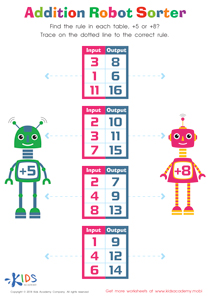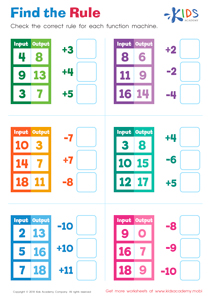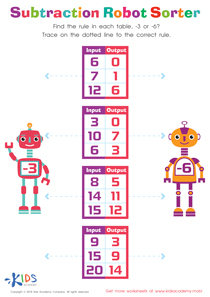# Math Lesson - What’s the Rule - Part 1, Grade 1

• ### Activity 1 / Addition Robot Sorter WorksheetUsing this tracing paper worksheet, your child will have a better understanding of the rules of addition. The robots on both sides of the table have the signs +8 and +5. You and your child must figure out which equation completes the data in the each table. Carefully trace on the dotted lines to the correct rule. Help your young ones have a better understanding of math and adding equations with this traceable worksheet.

• ### Activity 3 / Find the Rule WorksheetIn elementary math, a function machine is a diagram that contains an input that applies a math rule, like a set operation of addition or subtraction, to create an output, or the answer. This means that each graph contains various numbers for input and output, but they are tied together by a common rule. Essentially, children are examining the diagram for a common relationship between each input and output value. Use this vivid worksheet to help your child analyze the function machines to find the addition or subtraction rule that is being used for each!

• ### Activity 4 / Subtraction Robot Sorter WorksheetFinding patterns and looking for relationships between numbers are essential skills for math mastery! Help your child get the practice he or she needs with the assistance of delightful robot friends! Look carefully at each function machine. Is the rule minus 3 or minus 6? For each diagram, see if the relationship between each number can be found using either rule. When the pattern is found, simply trace the dotted line to the side of the robot containing the correct rule!

?